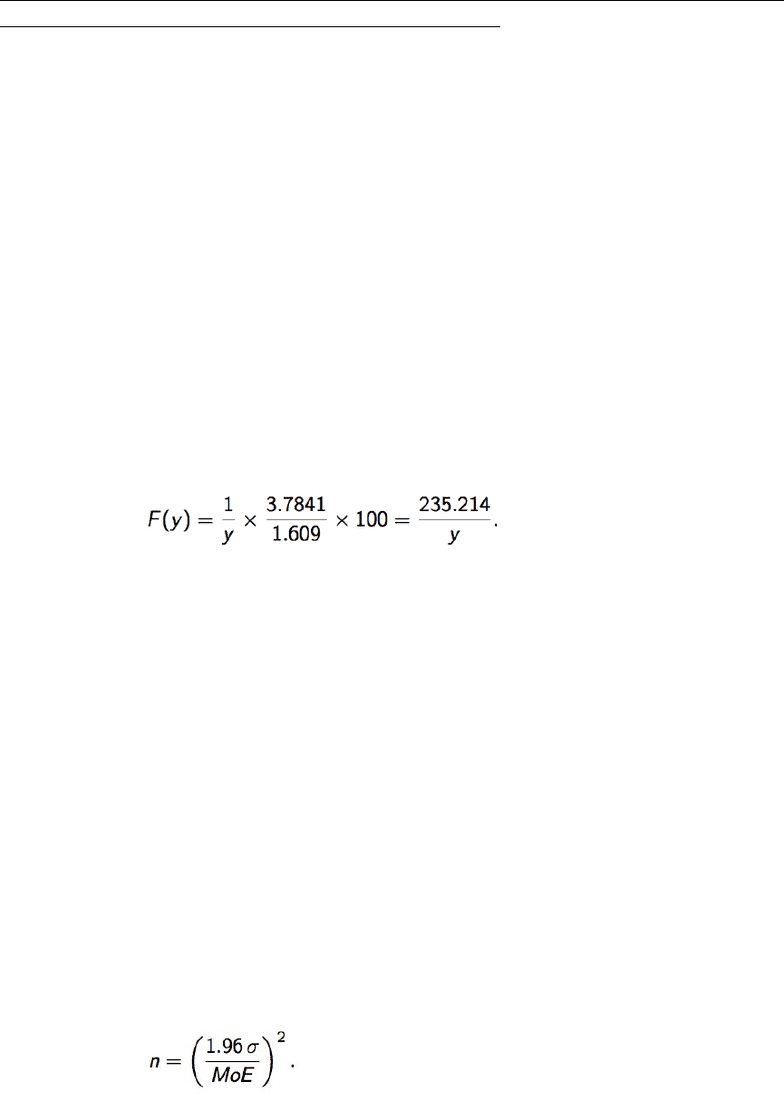Class Notes (1,100,000)
US (480,000)
UPenn (2,000)
STAT (70)
Lecture 18

# STAT 101 Lecture Notes - Lecture 18: Confidence Interval, Sample Size Determination, Odds RatioPremium

Department
Statistics
Course Code
STAT 101
Professor
Richard Waterman
Lecture
18

This preview shows half of the first page. to view the full 3 pages of the document.Stat 101 - Introduction to Business Statistics - Lecture 18: Interpreting Confidence
Intervals, Finding Sample Size, & Hypothesis Testing
Interpreting a Confidence Interval
● Correct:
95% of intervals created according to this procedure are expected to contain µ.
● Incorrect:
There is a 95% probability that µ lies in the interval.
Manipulating Confidence Intervals
You are allowed to transform the ends of the confidence interval to obtain a new
confidence interval for the transformed parameter
However transformation must be monotone though -- which means if the
relationship function doesn’t just increase or decrease
Ex) You have a 95% CI for the average miles per gallon for cars: (17.42, 18.230). Find a
95% CI for fuel economy when it is measured in liters per 100 kilometers. The transform
from MPG to ltrs./100km
The interval on the transformed scale is {F(18.23), F(17.42)} = (12.903
ltrs./100km, 13.503 ltrs./100km).
Ex) In many medical settings clinicians like to report results in terms of odds ratios rather
than probabilities. If the probability is given by p then the odds ratio is p/(1 − p). A study
has found the 95% confidence interval for p to be (0.35, 0.38). Find the 95% CI for the
odds ratio.
(0.35/0.65, 0.38/0.62) = (0.538, 0.613).
Sample Size
Must consider:
What confidence level you want (say 95%).
You need an estimate for σ.
What Margin of Error (MoE) you want.
Margin of error is the distance from the center to the edge of the interval
Given by MoE = 1.96 / n σ
Sample size: